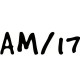Economics Notes (Class 12)

CBSE Class 12 Economics Microeconomics Chapter 3 Production and CostsWritten by
Rate this post

Production and CostsProduction and Costs

Important Notes

1. Production It is the transformation of resources into commodities.

2. Production Function Production function studies the functional relationship between physical input and physical output.
Y =F(L.K)
Here Y =Production, L = Labour, K = Capital.

3. Total Product It is the sum total of output produced by all units of labour.
TP= APx L
Here. TP = Total product, AP = Product per unit of labour
L = Units of labour

4. Marginal Product It is the change in total production as a result of a unit change in input of a variable factor (ΔL)
ΔTP = Change in total production
MP= TPn -TPn-1
or
MP=.ΔTP/ΔL

5. Average Product It is per unit production of the variable factor
AP= TP/L
Here, AP =Average product
TP = Total product
L= Labour

6. Short run The time period during which a firm, in order to make changes in its production can change only in its variable factors but not in its fixed factor, is termed as the short run.

7. Long run The time period in which a firm can change all the factors of production is termed as a long run. In the long period, a firm can change its scale of the plant also.

8. Law of Diminishing Marginal Product The law states that with the increase in variable factor, keeping all other factors constant the marginal product of the variable factor diminishes after a certain level of production. Reason for operating of law:

(i) Optimum combination
(ii) Change in factor combinations

9. Law of Variable Proportion The law states that with the increase in a variable factor, keeping another factor constant, initially the marginal product rises but after reaching a certain level of employment it starts declining.
Three stages of the law
(i) Increasing returns
(ii) Diminishing returns
(iii) Negative returns

10. Returns to Scale When producers change all the factors of production in the same production, the proportional relationship between output and factor inputs is known as returns to scale.
(i) Constant Returns to Scale When a proportional increase in all inputs results in an increase in output by the same proportion is called constant returns to scale.
(ii) Increasing Returns to Scale IRS holds when the proportional increase in all inputs results in an increase in output by more than the proportion.
(iii) Decreasing Returns to Scale DRS holds when proportional in all inputs results in an increase in output by less than the proportion.

11. Cost Function The functional relationship between cost and quantity produced is termed as the cost function.
C= F(Qx)
Here, C= Production – Cost
Qx = Quantity produced of x goods

12. Cost of Production Cost is the expenditure incurred by the producers on the purchase of factor inputs such and land, labour capital etc, non-factor inputs such as raw material. fuel etc.

13. Explicit Cost The cost of those inputs whose payment is made to the outside of the firm. It is an accounting cost.

14. Implicit Cost The cost of self-owned inputs used in the production process is called implicit cost.  e.g.. rent or own land, the interest of own Implicit etc.

15. Total Cost (TC) It is the sum total of fixed cost and variable cost corresponding to a given level of output.
‘I’C = TFC + TVC
Here, TFC = Total Fixed Cost
‘I’VC = Total Variable Cost
TC = Total Cost

16. Total Fixed Cost (TFC) TFC arc the costs that are incurred on fixed factor inputs and do not vary with the output. e.g., Rent of factory, Interest on bonds,
TFC = Quantities of the fixed productive services x Factor price
or TFC=TC-TVC

17. Total Variable Cost The costs that are incurred on variable factor inputs and very directly with the output are called total variable costs.
e.g., Raw material, fuel, electric power.
TVC= Quantities of the variable productive service x Factor price
or TVC= TC – TFC

18. Average Cost (AC) Average cost is the cost per unit of output produced.
AC= TC/Q ; Q= Units of output
AC=AFC+ AVC
AFC = Average Fixed Cost
AVC =Average Variable Cost

19. Average Fixed Cost Total fixed cost per unit of output incurred to a firm may be defined as average fixed cost. APC= TFC/Q

20. Averages Variable Cost (AVC) Total variable cost. per unit of output incurred to a firm is defined as the average variable cost. AFC= TVC/Q

21. Marginal Cost (MC) It is the additional cost owing to the production of an additional unit of output.  MCn =TCn -TCn-1 Since additional cost can only be the variable cost.

22. Shape of Curves
(i) AC, AVC, MC curve U shaped due to the law of returns to
the factor.
(ii) TFC curve straight  line || to X-axis.
(iii) TC and TVC curve upward sloping.
(iv) AFC curve downward rectangular hyperbola.